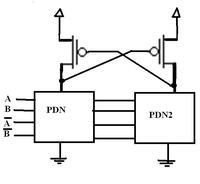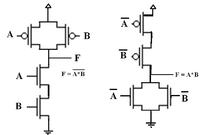# [SOLVED]simple Doubt about static CMOS logic

Status
Not open for further replies.

#### palmeiras

##### Full Member level 6
Hi Guys,
Please, could you clarify me the following confusion regarding Static CMOS logic?

In the Rabaey book, it is said that "Static CMOS gate is naturally inverting and the realization of a noninverting Boolean function (such as AND , OR, XOR) in a single stage is not possible."

I don’t completely understand the above explanation when I think that it is possible to design noninverting gates using STATIC CMOS Logic (for instance, the AND gate as shown in figure 1).

1- What is the message behind the above sentence?

2- In addition, what is the advantage of DCVSL (Differential Cascode Voltage Switch logic) compared to the traditional Static logic? why? Please, see figure 2.

Thank you very much,
Regards,When you input 1, the effect of input 1 shows up as 0 because logic 1 turns on N mos that is grounded and turns off P mos that is tied to VDD. The exact opposite to input 0. That's inverting the input.

As for AND gate you pointed to, it already has inputs inverted and doesn't apply to the case the book refers to since it's not a single stage logic.

Last edited:
•palmeiras

### palmeiras

Points: 2
Hi lostinxlation,

In standard static CMOS, please see that, the function realized is F' (compliment of F). If you want to realize F, then you have to take compliment of that, apply de morgan's theorem and then realize it. Eg, if you want to realize F= A.B, then take compliment. You'll get F'= A'+ B'. Then realize this function in the nmos pull down network and its compliment in the PMOS pull up network to realize the and gate.

What he means is, if a function F = function(A,B,C) is given, you can either realize F as a function of A',B' , C' or realize F' as a function of A,B, C.

regarding DCVSL, it eliminates the huge number of PMOS devices required and makes the circuit capable of a highe speed of operation. (since PMOS has holes as the charge carriers, they make the circuit slower as electrons are almost 3 times faster than holes.). Also, they remove the huge load capacitance offered by PMOS and this reduces loading to an appreciable extent. Both these advantages are also offered by ratioed logic, but in ratioed logic, the output voltage when logic high is less than Vdd. Here, since we have a positive feedback, the Voh will be the supply voltage..

•palmeiras

Points: 2